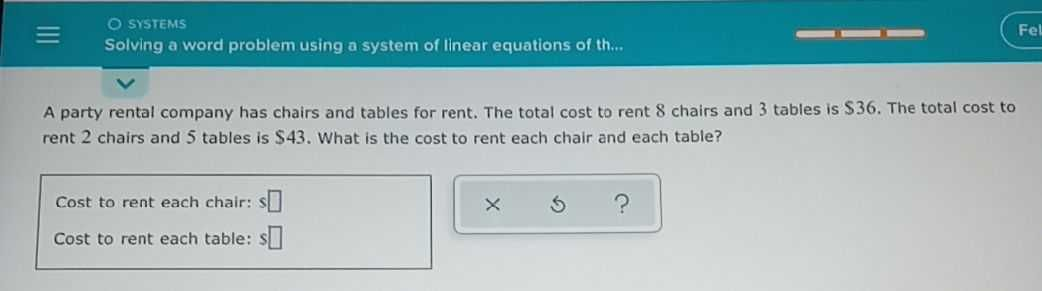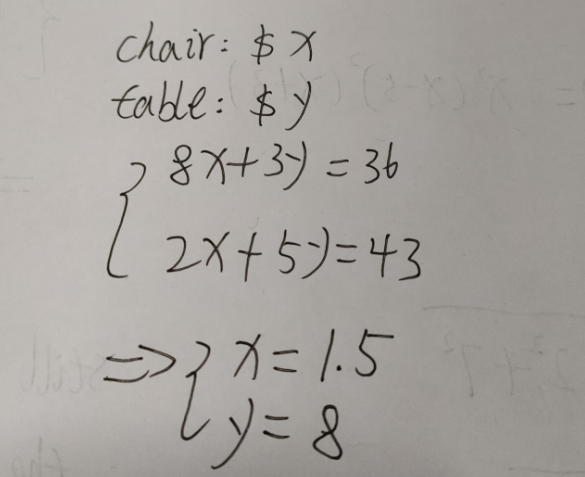### ¿Todavía tienes preguntas de matemáticas?

Pregunte a nuestros tutores expertos
Algebra
PreguntaA party rental company has chairs and tables for rent. The total cost to rent $$8$$ chairs and $$3$$ tables is $$\ 36$$ . The total cost to rent $$2$$ chairs and $$5$$ tables is $$543$$ . What is the cost to rent each chair and each table?